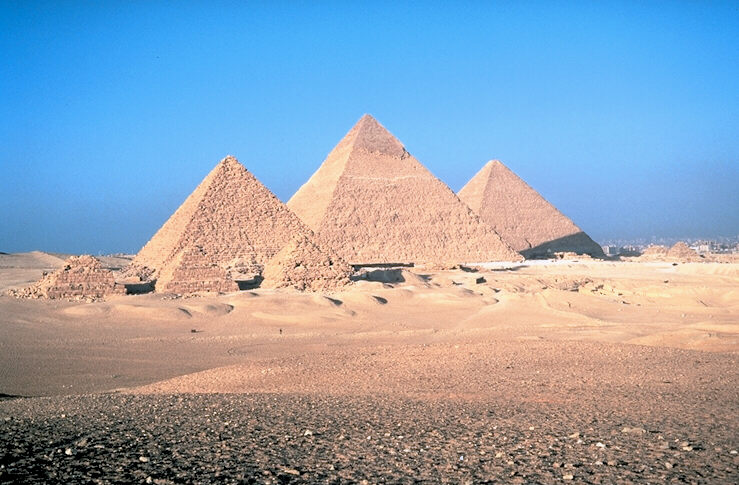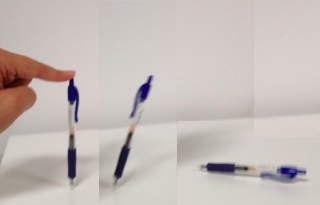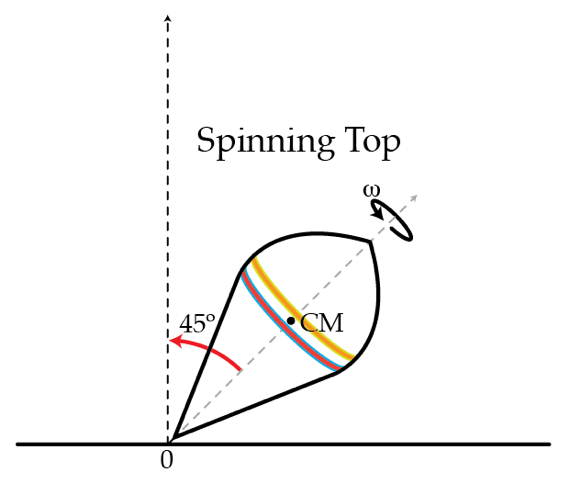Classical Mechanics

# Moment of Inertia: Level 3-5 ChallengesAs we saw in the previous problem, whomever worked on the pyramid moved a tremendous amount of stone from the quarries. Let's ignore the effort it took to get the stone from the quarry to the pyramid site, since we don't know the distance, how far they could travel by water vs. cart, etc. and just look at how much effort it took to lift that mass off the ground. To answer this question, we need to know the height of the center of mass of a uniform pyramid with the dimensions of the great pyramid: 150 m high and 230 m on a side. For such a pyramid, how high off the ground is the center of mass in m?I assume that you work through these problems using some sort of writing utensil. Take your pen, balance it on its tip, and let go. It falls over. How fast in m/s is the other end of the pen going when it hits the table, assuming the tip doesn't slip? Take the pen to be a uniform one dimensional rod of length 15 cm.

A picture is attached to a wall by two nails in the upper corners (these nails are at the very corners and also at the same height). The picture is wider horizontally than it is tall. Suddenly, one of the nails comes loose and so loses contact with the wall. If right after that moment, the y-component of the force acting on the picture by the other nail (still in contact with the wall) is unchanged, find the ratio of the horizontal width to the vertical height of the picture.

Details and assumptions

• The acceleration of gravity is $-9.8~\mbox{m/s}^2$.

Consider a rectangular sheet of metal with width $W=2~\mbox{m}$ and length $L=5~\mbox{m}$. The sheet is in the x-y plane, with the origin right in the geometric middle of the sheet. The x-axis is parallel to the short edge, while the y-axis is parallel to the long edge. The moment of inertia about the z-axis is $I_z=10~\mbox{kg}\cdot\mbox{m}^2$ and the moment of inertia about an axis that passes diagonally through the sheet (i.e. corner to corner) in the xy plane is $I=5~\mbox{kg}\cdot\mbox{m}^2$. What is the moment of inertia about the y-axis in $\mbox{kg}\cdot\mbox{m}^2$?

A top of mass $m=0.5~\mbox{kg}$ rotates with angular speed $\omega=350~\mbox{rad/s}$ around its axis which is tilted by a (constant) angle of $\theta=45^{\circ}$ as shown in the figure. The moment of inertia with respect to the top's axis is $I=2 ~\mbox{g} \cdot\mbox{m}^{2}$ and the distance from the tip of the top to the center of mass is $l=10~\mbox{cm}.$ Determine the force of friction $f$ in Newtons acting on the tip of the top.×